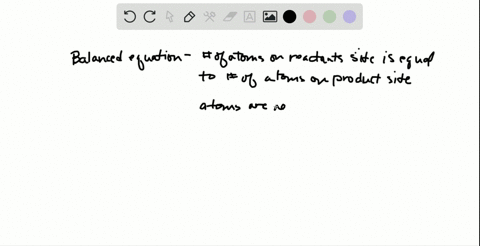🎉 Join all of us LIVE at 5PM PST and see if you are the lucky winner for this week's \$1500 Sweepstakes. 🎉Join and watch the live drawing!### How does a balanced chemical equation apply the l…

03:07University of Maine
Problem 52

# What three types of information does a balanced chemical equation provide? How?

## Discussion

You must be signed in to discuss.

## Video Transcript

a balanced chemical equation provides three things about a chemical reaction. The first is that it tells you which react INTs combine to make which product, so the first thing a balanced chemical equation tells us is the identity of the reactant and products. So in the example equation above, I know that magnesium will be reacting with oxygen to form magnesium oxide. The second thing that a balanced chemical equation tells us is not only what the reactions are, but what physical state of the reactions and products. So, for example, in the equation above the sub script s indicates a solid. The subscript G indicates that I'm using a gas form of oxygen. Other states you might see include the L for liquid and sometimes a cube print a quist solution. The final information found in a balanced equation are the mole ratios, or the quantity is needed in order for the reaction to form the product desired. We call these the mole ratios, and these are the numbers called cowfish is that are in front of each substance. If there's no number, the ratio is one. So this equation tells me I need two moles of magnesium to react with one more of oxygen, and I will form two moles of magnesium oxide